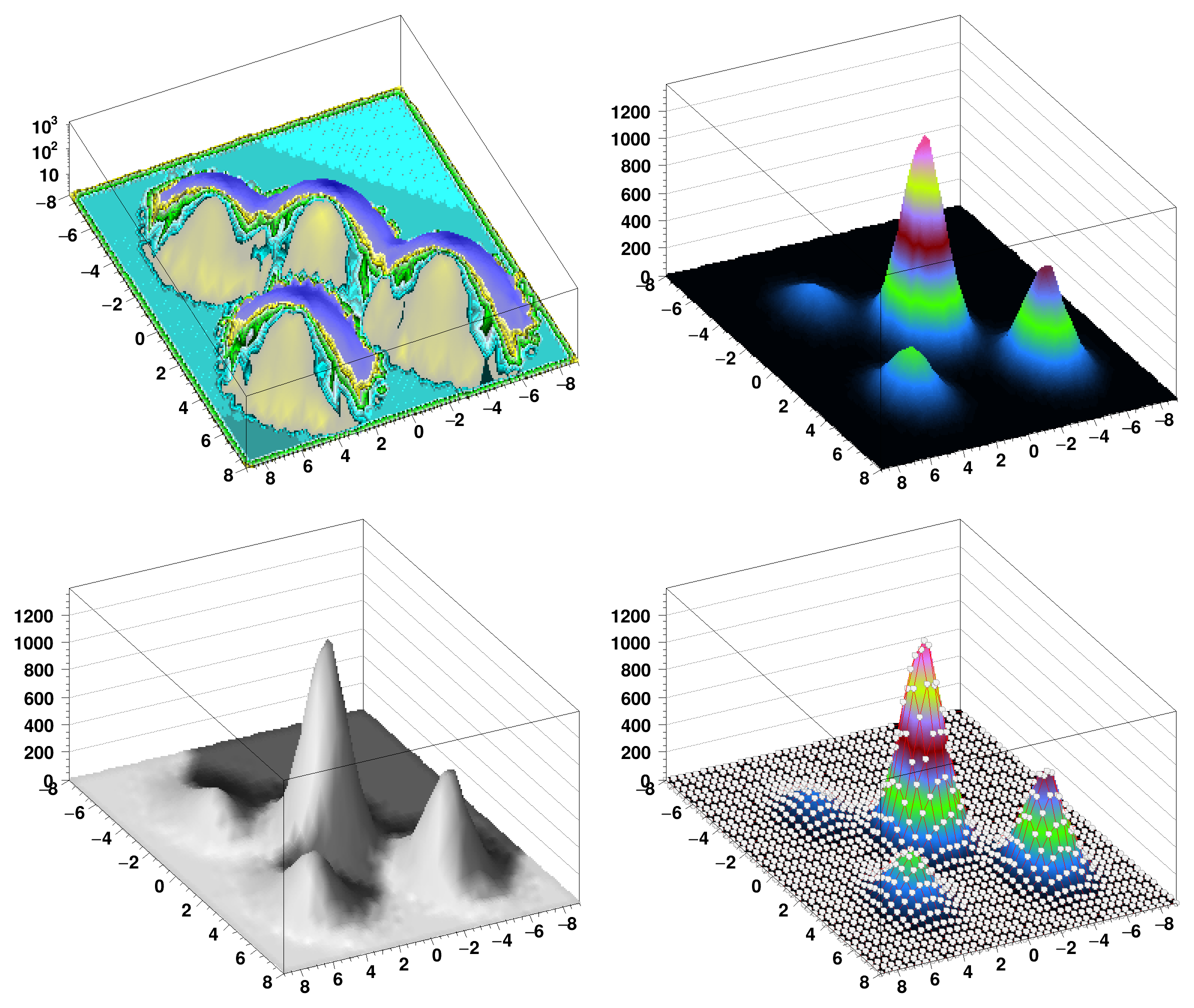ROOT   Reference Guide
Searching...
No Matches
spectrumpainter.C File Reference

## Detailed DescriptionExamples showing how to use TSpectrum2Painter (the SPEC option)void spectrumpainter()
{
TH2 *h2 = new TH2F("h2","h2",40,-8,8,40,-9,9);
Float_t px, py;
for (Int_t i = 0; i < 50000; i++) {
gRandom->Rannor(px,py);
h2->Fill(px,py);
h2->Fill(px+4,py-4,0.5);
h2->Fill(px+4,py+4,0.25);
h2->Fill(px-3,py+4,0.125);
}
TCanvas *c1 = new TCanvas("c1","Illustration of 2D graphics",10,10,800,700);
c1->Divide(2,2);
c1->cd(1);
h2->Draw("SPEC dm(2,10) zs(1)");
c1->cd(2);
h2->Draw("SPEC dm(1,10) pa(2,1,1) ci(1,4,8) a(15,45,0)");
c1->cd(3);
h2->Draw("SPEC dm(2,10) pa(1,1,1) ci(1,1,1) a(15,45,0) s(1,1)");
c1->cd(4);
h2->Draw("SPEC dm(1,10) pa(2,1,1) ci(1,4,8) a(15,45,0) cm(1,15,4,4,1) cg(1,2)");
}
int Int_t
Definition RtypesCore.h:45
float Float_t
Definition RtypesCore.h:57
R__EXTERN TRandom * gRandom
Definition TRandom.h:62
The Canvas class.
Definition TCanvas.h:23
void Draw(Option_t *option="") override
Draw this histogram with options.
Definition TH1.cxx:3060
2-D histogram with a float per channel (see TH1 documentation)}
Definition TH2.h:257
Service class for 2-D histogram classes.
Definition TH2.h:30
Int_t Fill(Double_t) override
Invalid Fill method.
Definition TH2.cxx:347
virtual void Rannor(Float_t &a, Float_t &b)
Return 2 numbers distributed following a gaussian with mean=0 and sigma=1.
Definition TRandom.cxx:500
return c1
Definition legend1.C:41

Definition in file spectrumpainter.C.